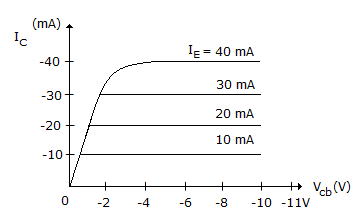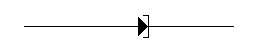Courses

# Test: Electronic Devices And Circuits - 7

## 25 Questions MCQ Test Electronic Devices | Test: Electronic Devices And Circuits - 7

Description
This mock test of Test: Electronic Devices And Circuits - 7 for Electrical Engineering (EE) helps you for every Electrical Engineering (EE) entrance exam. This contains 25 Multiple Choice Questions for Electrical Engineering (EE) Test: Electronic Devices And Circuits - 7 (mcq) to study with solutions a complete question bank. The solved questions answers in this Test: Electronic Devices And Circuits - 7 quiz give you a good mix of easy questions and tough questions. Electrical Engineering (EE) students definitely take this Test: Electronic Devices And Circuits - 7 exercise for a better result in the exam. You can find other Test: Electronic Devices And Circuits - 7 extra questions, long questions & short questions for Electrical Engineering (EE) on EduRev as well by searching above.
QUESTION: 1

Solution:
QUESTION: 2

Solution:
QUESTION: 3

### In CE configuration, the output characteristics of a bipolar junction transistor is drawn between

Solution:
QUESTION: 4

Assertion (A): The reverse current in a p-n junction is nearly constant.

Reason (R): The reverse breakdown voltage of a p-n diode depends on the extent of doping.

Solution:
QUESTION: 5

An n type semiconductor is illuminated by a steady flux of photons with energy greater than the band gap energy. The change in conductivity Δσ obeys which relation?
[ Here, e is the electron charge, μn electron mobility, μp hole mobility, Δnp) is the excess electron (hole) density ].

Solution:
QUESTION: 6

Resistivity of metals is expressed in terms of

Solution:
QUESTION: 7

Figure shows characteristics curves for bipolar transistor. These curves areSolution:
QUESTION: 8

Zener diode is invariably used with

Solution:
QUESTION: 9

The relation between plate current and plate voltage of a vacuum diode is called

Solution:
QUESTION: 10

Given that the band gap of cadmium sulphide is 2.5 eV, the maximum photon wavelength, for e--hole pair generation will be

Solution:
QUESTION: 11

The maximum rectification efficiency in case of full wave rectifier is

Solution:
QUESTION: 12

For a full wave bridge rectifier supplied with 50 Hz a.c., the lowest ripple frequency will be

Solution:
QUESTION: 13

When an electron rises through a potential of 100 V it will acquired an energy of

Solution:
QUESTION: 14

Which one of the following gain equations is correct for a MOSFET common-source amplifier?
(gm is mutual conductance, and RD is load resistance at the drain)

Solution:
QUESTION: 15

If 1 kVA transformer is used for all of the following rectifiers, the d.c. power availability will be least in case of

Solution:
QUESTION: 16

Which of the following is the ferric electric material?

Solution:
QUESTION: 17

The amount of time between the creation and disappearance of a hole in an intrinsic semiconductor material is called

Solution:
QUESTION: 18

In photo electric emission, the threshold frequency f0, work function Uw, and Planck's constant h are related as

Solution:
QUESTION: 19

In which n type device does p substrate extend upto silicon dioxide layer?

Solution:
QUESTION: 20

In a N-type semi-conductor, the concentration of minority carriers is mainly depends on

Solution:
QUESTION: 21

The band gap of Si at room temperature is

Solution:
QUESTION: 22

Spot the odd one out

Solution:
QUESTION: 23

Transition capacitance is associated with __________ and depletion capacitance is associated with __________ diodes.

Solution:
QUESTION: 24

Figure represents aSolution:
QUESTION: 25

If the energy gap of a semiconductor is 1.1 eV, then it would be.

Solution: